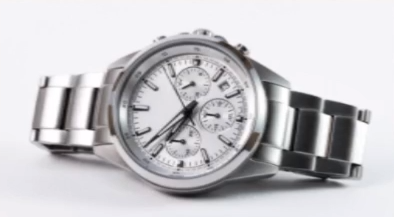What unit would you use to measure the mass of the following?What unit would you use to measure the mass of the following?What is likely to be the mass of the following?What is likely to be the mass of the following?